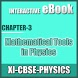# 11-PHY-Mathematical Tools in Physics THEORY EBOOKFree App

Rated 0.00/5 (0) —  Free Android application by Learn with Quiz

## About 11-PHY-Mathematical Tools in Physics THEORY EBOOK

Board: CBSE, GSEB, ICSE, ISC, International Board, Cambridge
Class: 11th - 12th Students, Engineering Students and Medical Students.

Physics (AIEEE, IIT-JEE Main) Atomic Structure Interactive e-Book Education application

Physics Online Quiz Apps; Chemistry Quizzes for Middle school and High School

Topic:
1. Elementary calculus
2. Constant quantity and variable quantity
3. Function
4. Limit of variable
5. Difference and Differential Coefficient
6. Basic Theorems on Differentiation
7. Different methods of differentiation
8. Differential coefficient of Trigonometrical functions
9. Derivatives of logarithmic and exponential functions
10. Derivatives of inverse trigonometric functions
11. Application of differentiation in Physics
12. Application of differentiation – Maxima and Minima
13. Integration
14. Fundamental Formulae of integration
15. Fundamental Theorem
16. Different Methods of Integration
17. Definite Integral
18. Geometrical meaning of definite integral

Free
downl.
Android
5.0+
For everyone
Android app

Oh snap! No comments are available for 11-PHY-Mathematical Tools in Physics THEORY EBOOK at the moment. Be the first to leave one!

RATING
0.05
0 users

5

4

3

2

1